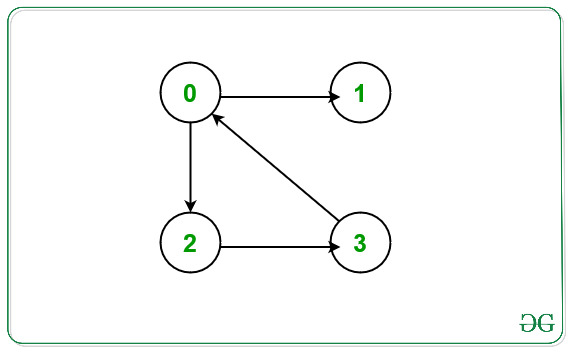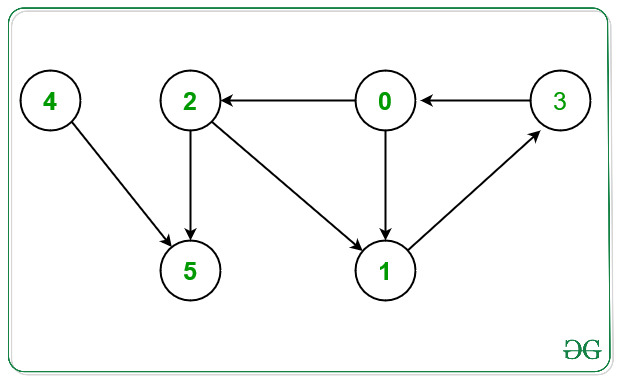Related Articles
Print Nodes which are not part of any cycle in a Directed Graph
• Last Updated : 16 Dec, 2020

Given a directed graph G N nodes and E Edges consisting of nodes valued [0, N – 1] and a 2D array Edges[] of type {u, v} that denotes a directed edge between vertices u and v. The task is to find the nodes that are not part of any cycle in the given graph G.

Examples:

Input: N = 4, E = 4, Edges[] = { {0, 2}, {0, 1}, {2, 3}, {3, 0} }
Output: 1
Explanation:From the given graph above there exists a cycle between the nodes 0 -> 2 -> 3 -> 0.
Node which doesn’t occurs in any cycle is 1.
Hence, print 1.

Input: N = 6, E = 7, Edges[] = { {0, 1}, {0, 2}, {1, 3}, {2, 1}, {2, 5}, {3, 0}, {4, 5}}
Output: 4 5
Explanation:From the given graph above there exists a cycle between the nodes:
1) 0 -> 1 -> 3 -> 0.
2) 0 -> 2 -> 1 -> 3 -> 0.
Nodes which doesn’t occurs in any cycle are 4 and 5.
Hence, print 4 and 5.

Naive Approach: The simplest approach is to detect a cycle in a directed graph for each node in the given graph and print only those nodes that are not a part of any cycle in the given graph.
Time Complexity: O(V * (V + E)), where V is the number of vertices and E is the number of edges.
Auxiliary Space: O(V)

Efficient Approach: To optimize the above approach, the idea is to store the intermediate node as a visited cycle node whenever any cycle in the given graph. To implement this part use an auxiliary array cyclePart[] that will store the intermediate cycle node while performing the DFS Traversal. Below are the steps:

• Initialize an auxiliary array cyclePart[] of size N, such that if cyclePart[i] = 0, then ith node doesn’t exist in any cycle.
• Initialize an auxiliary array recStack[] of size N, such that it will store the visited node in the recursion stack by marking that node as true.
• Perform the DFS Traversal on the given graph for each unvisited node and do the following:
• Now find a cycle in the given graph, whenever a cycle is found, mark the node in cyclePart[] as true as this node is a part of the cycle.
• If any node is visited in the recursive call and is recStack[node] is also true then that node is a part of the cycle then mark that node as true.
• After performing the DFS Traversal, traverse the array cyclePart[] and print all those nodes that are marked as false as these nodes are not the part of any cycle.

Below is the implementation of the above approach:

## C++

 `// C++ program for the above approach`   `#include ` `using` `namespace` `std;`   `class` `Graph {`   `    ``// No. of vertices` `    ``int` `V;`   `    ``// Stores the Adjacency List` `    ``list<``int``>* adj;` `    ``bool` `printNodesNotInCycleUtil(` `        ``int` `v, ``bool` `visited[], ``bool``* rs,` `        ``bool``* cyclePart);`   `public``:` `    ``// Constructor` `    ``Graph(``int` `V);`   `    ``// Member Functions` `    ``void` `addEdge(``int` `v, ``int` `w);` `    ``void` `printNodesNotInCycle();` `};`   `// Function to initialize the graph` `Graph::Graph(``int` `V)` `{` `    ``this``->V = V;` `    ``adj = ``new` `list<``int``>[V];` `}`   `// Function that adds directed edges` `// between node v with node w` `void` `Graph::addEdge(``int` `v, ``int` `w)` `{` `    ``adj[v].push_back(w);` `}`   `// Function to perform DFS Traversal` `// and return true if current node v` `// formes cycle` `bool` `Graph::printNodesNotInCycleUtil(` `    ``int` `v, ``bool` `visited[],` `    ``bool``* recStack, ``bool``* cyclePart)` `{`   `    ``// If node v is unvisited` `    ``if` `(visited[v] == ``false``) {`   `        ``// Mark the current node as` `        ``// visited and part of` `        ``// recursion stack` `        ``visited[v] = ``true``;` `        ``recStack[v] = ``true``;`   `        ``// Traverse the Adjacency` `        ``// List of current node v` `        ``for` `(``auto``& child : adj[v]) {`   `            ``// If child node is unvisited` `            ``if` `(!visited[child]` `                ``&& printNodesNotInCycleUtil(` `                       ``child, visited,` `                       ``recStack, cyclePart)) {`   `                ``// If child node is a part` `                ``// of cycle node` `                ``cyclePart[child] = 1;` `                ``return` `true``;` `            ``}`   `            ``// If child node is visited` `            ``else` `if` `(recStack[child]) {` `                ``cyclePart[child] = 1;` `                ``return` `true``;` `            ``}` `        ``}` `    ``}`   `    ``// Remove vertex from recursion stack` `    ``recStack[v] = ``false``;` `    ``return` `false``;` `}`   `// Function that print the nodes for` `// the given directed graph that are` `// not present in any cycle` `void` `Graph::printNodesNotInCycle()` `{`   `    ``// Stores the visited node` `    ``bool``* visited = ``new` `bool``[V];`   `    ``// Stores nodes in recursion stack` `    ``bool``* recStack = ``new` `bool``[V];`   `    ``// Stores the nodes that are` `    ``// part of any cycle` `    ``bool``* cyclePart = ``new` `bool``[V];`   `    ``for` `(``int` `i = 0; i < V; i++) {` `        ``visited[i] = ``false``;` `        ``recStack[i] = ``false``;` `        ``cyclePart[i] = ``false``;` `    ``}`   `    ``// Traverse each node` `    ``for` `(``int` `i = 0; i < V; i++) {`   `        ``// If current node is unvisited` `        ``if` `(!visited[i]) {`   `            ``// Perform DFS Traversal` `            ``if` `(printNodesNotInCycleUtil(` `                    ``i, visited, recStack,` `                    ``cyclePart)) {`   `                ``// Mark as cycle node` `                ``// if it return true` `                ``cyclePart[i] = 1;` `            ``}` `        ``}` `    ``}`   `    ``// Traverse the cyclePart[]` `    ``for` `(``int` `i = 0; i < V; i++) {`   `        ``// If node i is not a part` `        ``// of any cycle` `        ``if` `(cyclePart[i] == 0) {` `            ``cout << i << ``" "``;` `        ``}` `    ``}` `}`   `// Function that print the nodes for` `// the given directed graph that are` `// not present in any cycle` `void` `solve(``int` `N, ``int` `E,` `           ``int` `Edges[])` `{`   `    ``// Initialize the graph g` `    ``Graph g(N);`   `    ``// Create a directed Graph` `    ``for` `(``int` `i = 0; i < E; i++) {` `        ``g.addEdge(Edges[i],` `                  ``Edges[i]);` `    ``}`   `    ``// Function Call` `    ``g.printNodesNotInCycle();` `}`   `// Driver Code` `int` `main()` `{` `    ``// Given Number of nodes` `    ``int` `N = 6;`   `    ``// Given Edges` `    ``int` `E = 7;`   `    ``int` `Edges[] = { { 0, 1 }, { 0, 2 }, ` `                       ``{ 1, 3 }, { 2, 1 }, ` `                       ``{ 2, 5 }, { 3, 0 }, ` `                                 ``{ 4, 5 } };`   `    ``// Function Call` `    ``solve(N, E, Edges);`   `    ``return` `0;` `}`

## Python3

 `# Python3 program for the above approach`   `class` `Graph:` `    `  `    ``# Function to initialize the graph` `    ``def` `__init__(``self``, V):` `        `  `        ``self``.V ``=` `V` `        ``self``.adj ``=` `[[] ``for` `i ``in` `range``(``self``.V)]` `    `  `    ``# Function that adds directed edges` `    ``# between node v with node w` `    ``def` `addEdge(``self``, v, w):` `    `  `        ``self``.adj[v].append(w);` `    `  `    ``# Function to perform DFS Traversal` `    ``# and return True if current node v` `    ``# formes cycle` `    ``def` `printNodesNotInCycleUtil(``self``, v, visited,recStack, cyclePart):` `    `  `        ``# If node v is unvisited` `        ``if` `(visited[v] ``=``=` `False``):` `        `  `            ``# Mark the current node as` `            ``# visited and part of` `            ``# recursion stack` `            ``visited[v] ``=` `True``;` `            ``recStack[v] ``=` `True``;` `    `  `            ``# Traverse the Adjacency` `            ``# List of current node v` `            ``for` `child ``in` `self``.adj[v]:` `            `  `                ``# If child node is unvisited` `                ``if` `(``not` `visited[child] ``and` `self``.printNodesNotInCycleUtil(child, visited,recStack, cyclePart)):` `                           `  `                    ``# If child node is a part` `                    ``# of cycle node` `                    ``cyclePart[child] ``=` `1``;` `                    ``return` `True``;` `    `  `                ``# If child node is visited` `                ``elif` `(recStack[child]):` `                    ``cyclePart[child] ``=` `1``;` `                    ``return` `True``;` `                    `  `        ``# Remove vertex from recursion stack` `        ``recStack[v] ``=` `False``;` `        ``return` `False``;` `    `  `    ``# Function that print the nodes for` `    ``# the given directed graph that are` `    ``# not present in any cycle` `    ``def` `printNodesNotInCycle(``self``):` `    `  `        ``# Stores the visited node` `        ``visited ``=` `[``False` `for` `i ``in` `range``(``self``.V)];` `    `  `        ``# Stores nodes in recursion stack` `        ``recStack ``=` `[``False` `for` `i ``in` `range``(``self``.V)];` `    `  `        ``# Stores the nodes that are` `        ``# part of any cycle` `        ``cyclePart ``=` `[``False` `for` `i ``in` `range``(``self``.V)]` `    `  `        ``# Traverse each node` `        ``for` `i ``in` `range``(``self``.V):` `        `  `            ``# If current node is unvisited` `            ``if` `(``not` `visited[i]):` `            `  `                ``# Perform DFS Traversal` `                ``if``(``self``.printNodesNotInCycleUtil(` `                        ``i, visited, recStack,` `                        ``cyclePart)):` `                        `  `                    ``# Mark as cycle node` `                    ``# if it return True` `                    ``cyclePart[i] ``=` `1``;` `    `  `        ``# Traverse the cyclePart[]` `        ``for` `i ``in` `range``(``self``.V):` `        `  `            ``# If node i is not a part` `            ``# of any cycle` `            ``if` `(cyclePart[i] ``=``=` `0``) :` `                ``print``(i,end``=``' '``)` `                    `  `# Function that print the nodes for` `# the given directed graph that are` `# not present in any cycle` `def` `solve( N, E, Edges):`   `    ``# Initialize the graph g` `    ``g ``=` `Graph(N);`   `    ``# Create a directed Graph` `    ``for` `i ``in` `range``(E):`   `        ``g.addEdge(Edges[i][``0``],` `                  ``Edges[i][``1``]);   `   `    ``# Function Call` `    ``g.printNodesNotInCycle();    ` `    `  `    ``# Driver Code` `if` `__name__``=``=``'__main__'``:` `    `  `    ``# Given Number of nodes` `    ``N ``=` `6``;` ` `  `    ``# Given Edges` `    ``E ``=` `7``;` ` `  `    ``Edges ``=` `[ [ ``0``, ``1` `], [ ``0``, ``2` `], ` `                       ``[ ``1``, ``3` `], [ ``2``, ``1` `], ` `                       ``[ ``2``, ``5` `], [ ``3``, ``0` `], ` `                                 ``[ ``4``, ``5` `] ];` ` `  `    ``# Function Call` `    ``solve(N, E, Edges);` `  `  `# This code is contributed by rutvik_56`

Output:

`4 5`

Time Complexity: O(V + E)
Space Complexity: O(V)

Attention reader! Don’t stop learning now. Get hold of all the important DSA concepts with the DSA Self Paced Course at a student-friendly price and become industry ready.

My Personal Notes arrow_drop_up
Recommended Articles
Page :